# 十七、聚类

## 凝聚聚类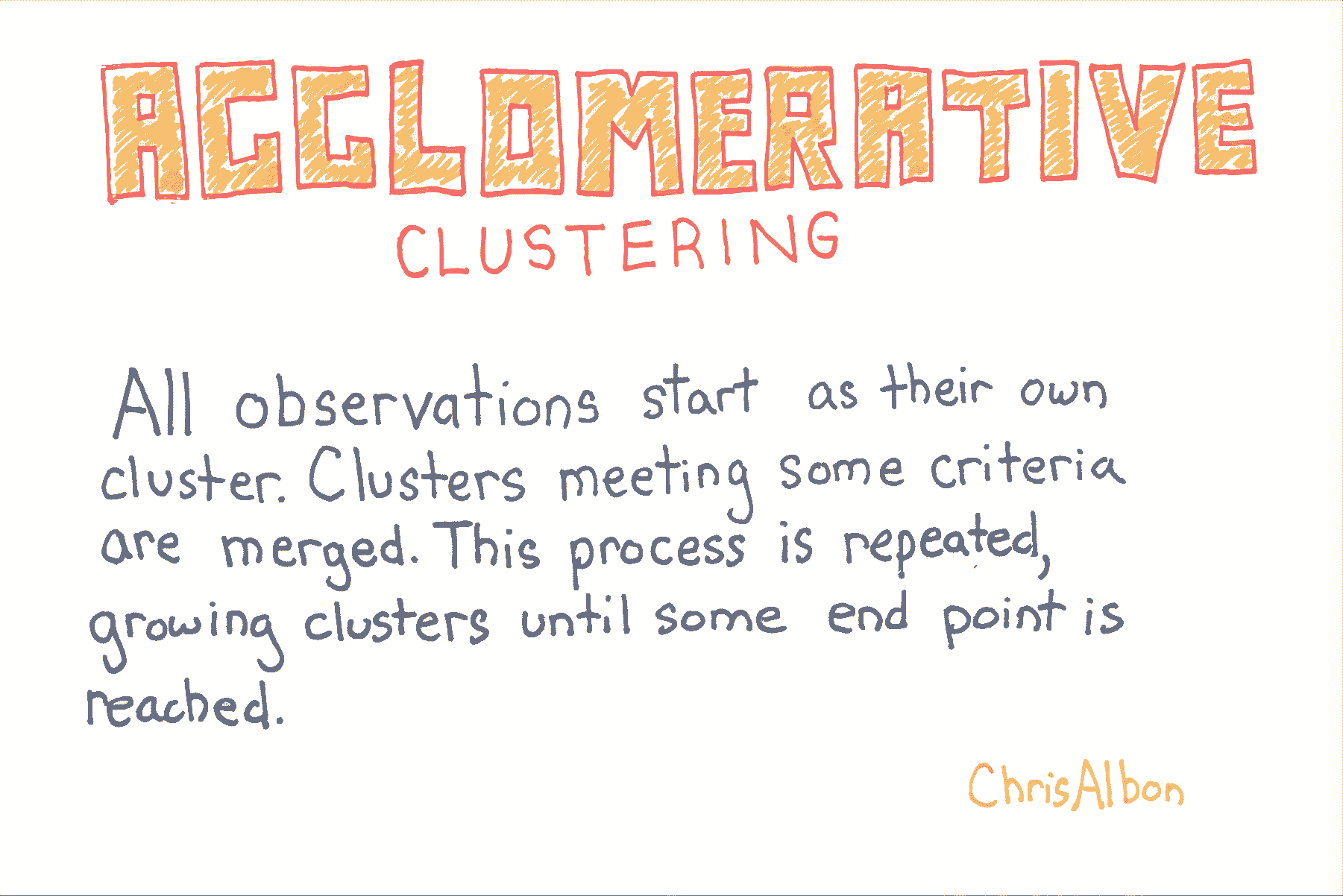``````# 加载库
from sklearn import datasets
from sklearn.preprocessing import StandardScaler
from sklearn.cluster import AgglomerativeClustering

# 加载数据
X = iris.data

# 标准化特征
scaler = StandardScaler()
X_std = scaler.fit_transform(X)
``````

``````# 创建聚类对象
affinity='euclidean',
n_clusters=3)

# 训练模型
model = clt.fit(X_std)

# 展示簇的成员
model.labels_

'''
array([1, 1, 1, 1, 1, 1, 1, 1, 1, 1, 1, 1, 1, 1, 1, 1, 1, 1, 1, 1, 1, 1, 1,
1, 1, 1, 1, 1, 1, 1, 1, 1, 1, 1, 1, 1, 1, 1, 1, 1, 1, 2, 1, 1, 1, 1,
1, 1, 1, 1, 0, 0, 0, 2, 0, 2, 0, 2, 0, 2, 2, 0, 2, 0, 0, 0, 0, 2, 2,
2, 0, 0, 0, 0, 0, 0, 0, 0, 0, 2, 2, 2, 2, 0, 0, 0, 0, 2, 0, 2, 2, 0,
2, 2, 2, 0, 0, 0, 2, 2, 0, 0, 0, 0, 0, 0, 2, 0, 0, 0, 0, 0, 0, 0, 0,
0, 0, 0, 0, 2, 0, 0, 0, 0, 0, 0, 0, 0, 0, 0, 0, 0, 0, 0, 0, 0, 0, 0,
0, 0, 0, 0, 0, 0, 0, 0, 0, 0, 0, 0])
'''
``````

## DBSCAN 聚类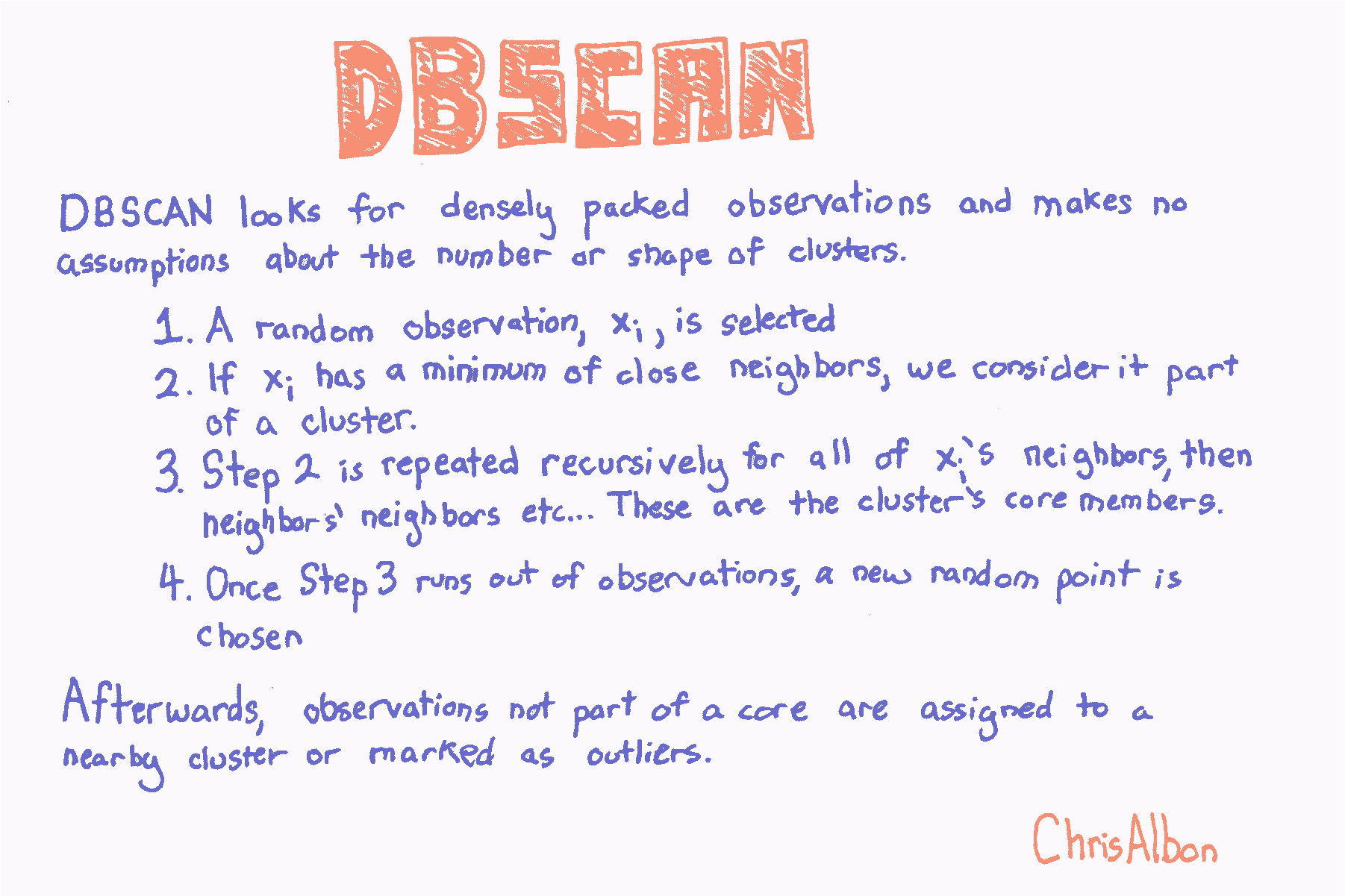``````# 加载库
from sklearn import datasets
from sklearn.preprocessing import StandardScaler
from sklearn.cluster import DBSCAN

# 加载数据
X = iris.data

# 标准化特征
scaler = StandardScaler()
X_std = scaler.fit_transform(X)
``````

`DBSCAN`有三个要设置的主要参数：

• `eps`: 观测到被认为是邻居的另一个观测的最大距离
• `min_samples`: 小于上面的`eps`距离的最小观测数量
• `metric`: `eps`使用的距离度量。 例如，`minkowski``euclidean`等（请注意，如果使用 Minkowski 距离，参数`p`可用于设置 Minkowski 度量的指数）

``````# 创建 DBSCAN 对象
clt = DBSCAN(n_jobs=-1)

# 训练模型
model = clt.fit(X_std)
``````

## 评估聚类

``````import numpy as np
from sklearn.metrics import silhouette_score
from sklearn import datasets
from sklearn.cluster import KMeans
from sklearn.datasets import make_blobs

# 生成特征矩阵
X, _ = make_blobs(n_samples = 1000,
n_features = 10,
centers = 2,
cluster_std = 0.5,
shuffle = True,
random_state = 1)

# 使用 k-means 来对数据聚类
model = KMeans(n_clusters=2, random_state=1).fit(X)

# 获取预测的类别
y_hat = model.labels_
``````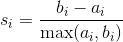``````# 评估模型
silhouette_score(X, y_hat)

# 0.89162655640721422
``````

## 均值移动聚类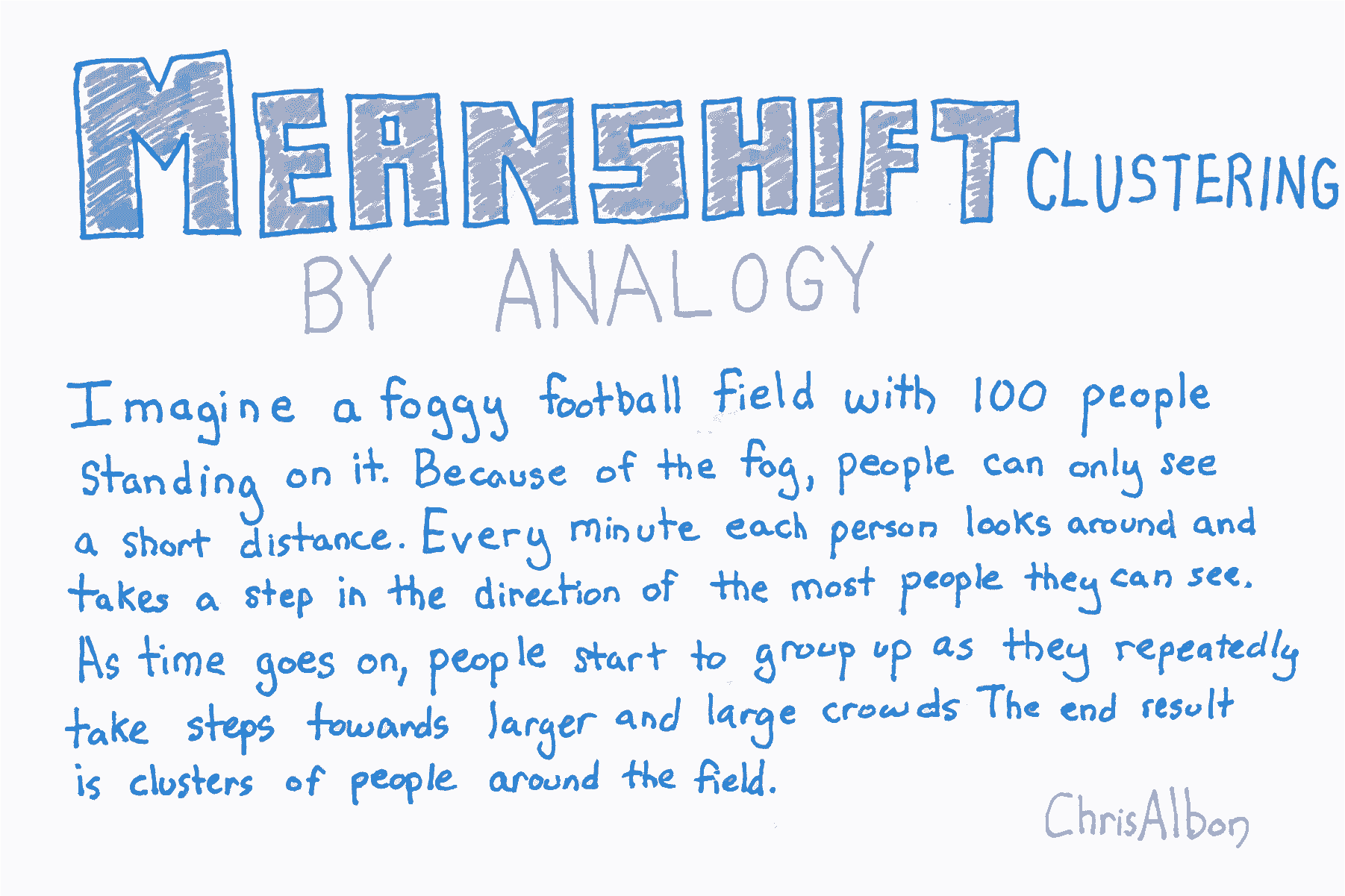``````# 加载库
from sklearn import datasets
from sklearn.preprocessing import StandardScaler
from sklearn.cluster import MeanShift

# 加载数据
X = iris.data

# 标准化特征
scaler = StandardScaler()
X_std = scaler.fit_transform(X)
``````

`MeanShift`有两个我们应该注意的重要参数。 首先，`bandwidth`设置区域（即观测核）半径，用于确定移动方向。 在我们的比喻中，带宽是一个人可以在雾中看到的距离。 我们可以手动设置此参数，但默认情况下会自动估算合理的带宽（计算成本会显着增加）。 其次，有时在均值移动中，观测核中没有其他观测结果。 也就是说，我们足球上的一个人看不到任何其它人。 默认情况下，`MeanShift`将所有这些“孤例”观测值分配给最近观测核。 但是，如果我们想要留出这些孤例，我们可以设置`cluster_all = False`，其中孤例观测标签为 -1。

``````# 创建 MeanShift 对象
clt = MeanShift(n_jobs=-1)

# 训练模型
model = clt.fit(X_std)
``````

## 小批量 KMeans 聚类

``````# 加载库
from sklearn import datasets
from sklearn.preprocessing import StandardScaler
from sklearn.cluster import MiniBatchKMeans

# 加载数据
X = iris.data

# 标准化特征
scaler = StandardScaler()
X_std = scaler.fit_transform(X)
``````

`MiniBatchKMeans``KMeans`的工作方式类似，有一个显着性差异：`batch_size`参数。 `batch_size`控制每批中随机选择的观测数。 批量越大，训练过程的计算成本就越高。

``````# 创建 KMeans 对象
clustering = MiniBatchKMeans(n_clusters=3, random_state=0, batch_size=100)

# 训练模型
model = clustering.fit(X_std)
``````

## KMeans 聚类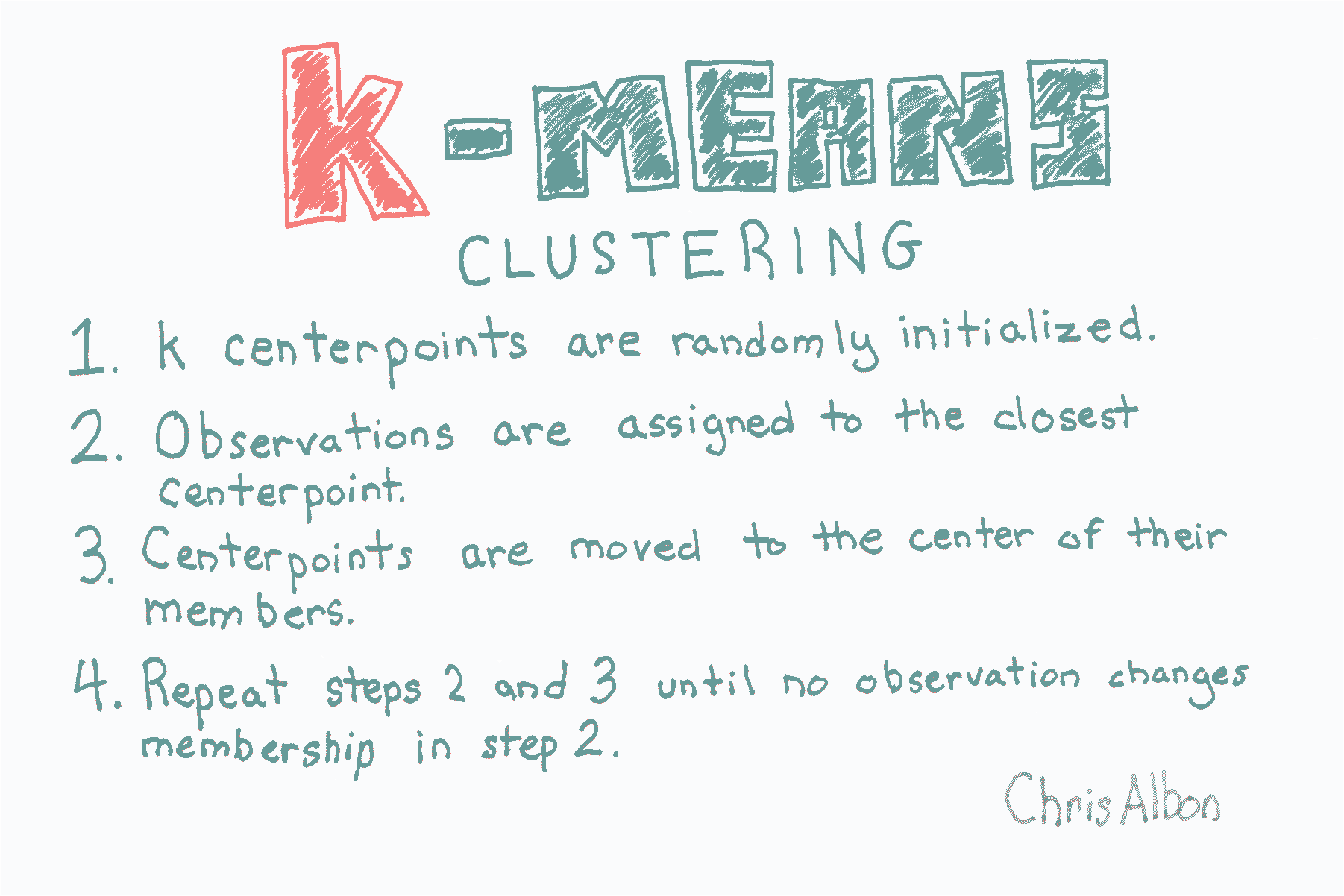``````# 加载库
from sklearn import datasets
from sklearn.preprocessing import StandardScaler
from sklearn.cluster import KMeans

# 加载数据
X = iris.data

# 标准化特征
scaler = StandardScaler()
X_std = scaler.fit_transform(X)

# 创建 KMeans 对象
clt = KMeans(n_clusters=3, random_state=0, n_jobs=-1)

# 训练模型
model = clt.fit(X_std)

# 查看预测类别
model.labels_

'''
array([1, 1, 1, 1, 1, 1, 1, 1, 1, 1, 1, 1, 1, 1, 1, 1, 1, 1, 1, 1, 1, 1, 1,
1, 1, 1, 1, 1, 1, 1, 1, 1, 1, 1, 1, 1, 1, 1, 1, 1, 1, 1, 1, 1, 1, 1,
1, 1, 1, 1, 0, 0, 0, 2, 2, 2, 0, 2, 2, 2, 2, 2, 2, 2, 2, 0, 2, 2, 2,
2, 0, 2, 2, 2, 2, 0, 0, 0, 2, 2, 2, 2, 2, 2, 2, 0, 0, 2, 2, 2, 2, 2,
2, 2, 2, 2, 2, 2, 2, 2, 0, 2, 0, 0, 0, 0, 2, 0, 0, 0, 0, 0, 0, 2, 2,
0, 0, 0, 0, 2, 0, 2, 0, 2, 0, 0, 2, 0, 0, 0, 0, 0, 0, 2, 2, 0, 0, 0,
2, 0, 0, 0, 2, 0, 0, 0, 2, 0, 0, 2], dtype=int32)
'''

# 创建新的观测
new_observation = [[0.8, 0.8, 0.8, 0.8]]

# 预测观测的类别
model.predict(new_observation)

# array(, dtype=int32)

# 查看簇中心
model.cluster_centers_

'''
array([[ 1.13597027,  0.09659843,  0.996271  ,  1.01717187],
[-1.01457897,  0.84230679, -1.30487835, -1.25512862],
[-0.05021989, -0.88029181,  0.34753171,  0.28206327]])
'''
``````